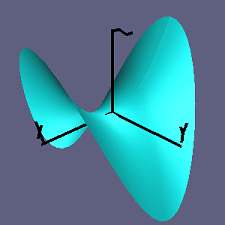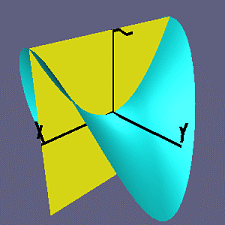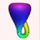Up: Curvatures

Principal Curvatures

Since we are going to consider C^2 surfaces we can start our study by considering a surface S which is the graph of a function with continuous second derivatives.
Let n denote a unit normal vector to S at a point p. And let p be the point in which the normal vector is pointing along the z-axis.

To study the curvature of S at p, we slice S by planes containing n and consider the curvature of the resulting curves.

Example: Let S the graph z=f(x,y) where f(x,y) = x^2-y^2 (the Saddle) and let p the point (0,0,0).

Slicing the surface with a plane:Movie showing different resulting curves when we rotate the plane by pi:

The curvatures of these resulting curves are called normal curvatures at p.

The maximum normal curvature k1 and the minimum normal curvature k2 are called principal curvatures.

In the movie, k1 is the curvature of the red curve and k2 is the curvature of the blue curve.

Up: Differential GeometryThe Geometry Center Home PageTopological Zoo Welcome Page